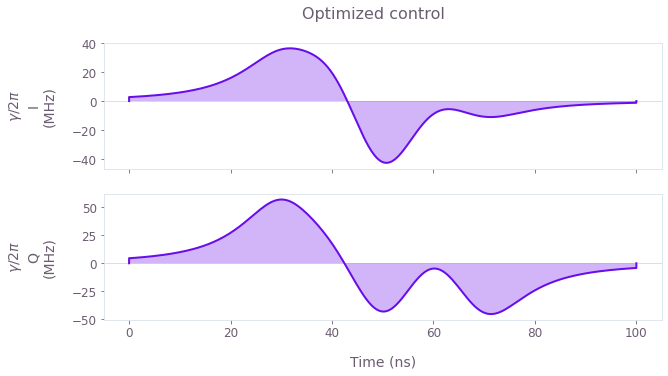•Boulder Opal
• User guides
• Optimize controls using user-defined basis functions

# How to optimize controls using user-defined basis functions

Create optimized controls using arbitrary basis functions

Boulder Opal exposes a highly-flexible optimization engine for general-purpose gradient-based optimization. The pulses can be described in terms of optimizable linear combinations from a set of user-defined basis functions, which can greatly reduce the dimensionality of the optimization search space. In this notebook we will use Lorentz functions, although the same technique has also seen success with other bases, for example Slepian functions. You can also read the related user guides showing how to find optimal pulses using a Fourier basis or a Hann series basis.

## Summary workflow

### 1. Define basis function for signal composition in the graph

The Boulder Opal optimization engine provides a library of operations for creating optimizable signals in a custom basis. In particular, you can create an analytical pulse by creating a node with the graph.identity_stf operation, which returns an STF representing the function $f(t) = t$, and then apply mathematical and arithmetic operations to it in order to generate more complex functions.

In the example below, the function custom_optimizable_superposition defines the composition of the pulse in terms of Lorentz functions.

### 2. Execute graph-based optimization

With the graph object created, you can run an optimization using the qctrl.functions.calculate_optimization function, providing the graph, the cost node, and the desired output nodes. The function returns the results of the optimization.

## Example: Robust optimization on a qutrit using a function superposition

In this example, we perform optimization for a robust single qubit Hadamard gate of a qutrit system while minimizing leakage out of the computational subspace. The system is described by the following Hamiltonian: \begin{equation} H(t) = \frac{\chi}{2} (a^\dagger)^2 a^2 + (1+\beta(t)) \left(\gamma(t) a + \gamma^*(t) a^\dagger \right) , \end{equation} where $\chi$ is the anharmonicity, $\gamma(t)$ is a complex time-dependent pulse, $\beta(t)$ is a small, slowly-varying stochastic amplitude noise process, and $a = |0 \rangle \langle 1 | + \sqrt{2} |1 \rangle \langle 2 |$.

In this example, we will parametrize the real and imaginary parts of the pulse, $\gamma(t) = \gamma_I(t) + i \gamma_Q(t)$, as a superposition of Lorentz functions, \begin{equation} \gamma_{I(Q)}(t) = \sum_{n=1}^N c^{I(Q)}_n \frac{\sigma^2}{(t - t_n)^2 + \sigma^2} , \end{equation} where $c^{I(Q)}_n$ are the different real-valued coefficients describing the parametrization, $\{t_n\}$ are the centers of the Lorentz functions, and $\sigma$ is their width. This is a good choice for implementation in bandwidth-limited hardware as it is composed of smooth functions that go to zero away from the choice of centers $\{t_n\}$.

Note that you can create a wide variety of analytical functions and superpositions using the different mathematical and arithmetic graph operations.

import matplotlib.pyplot as plt
import numpy as np

from qctrlvisualizer import get_qctrl_style, plot_controls

plt.style.use(get_qctrl_style())

from qctrl import Qctrl

# Start a Boulder Opal session.
qctrl = Qctrl()
# Define the function to generate the pulse components.
def custom_optimizable_superposition(graph, duration, coefficient_count, name):
"""
Create an STF optimizable superposition of Lorentzian functions.

Parameters
----------
graph : The graph where the signal will belong.
duration : The duration of the signal.
coefficient_count : The number of terms in the superposition.
name : The name of the Tensor node with the optimizable coefficients.

Returns
-------
Stf
An optimizable superposition of Lorentzian functions.
"""

# Define optimizable coefficients.
coefficients = graph.optimization_variable(
coefficient_count, lower_bound=-1, upper_bound=1, name=name
)

# Define Lorentz function parameters.
width = 0.1 * duration
centers = np.linspace(0.3, 0.7, coefficient_count) * duration

# Create Lorentz function superposition.
time = graph.identity_stf()
return graph.stf_sum(
[
coefficients[index] * width**2 / ((time - center) ** 2 + width**2)
for index, center in zip(range(coefficient_count), centers)
]
)

# Define target and projector matrices.
hadamard = np.array([[1.0, 1.0, 0], [1.0, -1.0, 0], [0, 0, np.sqrt(2)]]) / np.sqrt(2)
qubit_projector = np.diag([1.0, 0.0, 0.0])

# Define physical constraints
chi = -2 * np.pi * 300e6  # Hz
gamma_max = 2 * np.pi * 90e6  # Hz
segment_count = 200
duration = 100e-9  # s
sample_times = np.linspace(0, duration, segment_count)
coefficient_count = 5

# Create graph object.
graph = qctrl.create_graph()

# Define standard matrices.
a = graph.annihilation_operator(3)

# Create gamma(t) signal using custom basis.
gamma_i = custom_optimizable_superposition(
graph, duration, coefficient_count, name="gamma_i"
)
gamma_q = custom_optimizable_superposition(
graph, duration, coefficient_count, name="gamma_q"
)
gamma = gamma_max * (gamma_i + 1j * gamma_q)

# Discretize gamma to export and plot.
sample_gamma = graph.discretize_stf(
stf=gamma, duration=duration, segment_count=segment_count, name=r"$\gamma$"
)

# Create anharmonicity term.

# Create drive term.
drive = graph.hermitian_part(2 * gamma * a)

# Create target operator in qubit subspace.
target_operator = graph.target(
)

# Create infidelity.
infidelity = graph.infidelity_stf(
hamiltonian=anharmonicity + drive,
target=target_operator,
sample_times=sample_times,
noise_operators=[drive],
name="infidelity",
)

# Run the optimization.
optimization_result = qctrl.functions.calculate_optimization(
graph=graph,
cost_node_name="infidelity",
output_node_names=[r"$\gamma$", "gamma_i", "gamma_q"],
optimization_count=4,
)

# Retrieve results and plot optimized pulse.
coefficients_i = gamma_max * optimization_result.output.pop("gamma_q")["value"]
coefficients_q = gamma_max * optimization_result.output.pop("gamma_i")["value"]

print(f"\nOptimized cost: {optimization_result.cost:.3e}")
print("Optimized coefficients:")
np.set_printoptions(precision=3)
print("\tI:", coefficients_i)
print("\tQ:", coefficients_q)

plot_controls(optimization_result.output, smooth=True, polar=False)
plt.suptitle("Optimized control")
plt.show()
Your task calculate_optimization (action_id="1599308") has started.

Optimized cost: 2.683e-04
Optimized coefficients:
I: [ 3.735e+08  1.536e+08 -5.246e+08  3.596e+08 -3.902e+08]
Q: [ 1.854e+08  2.518e+08 -4.930e+08  1.655e+08 -8.825e+07]This notebook was run using the following package versions. It should also be compatible with newer versions of the Q-CTRL Python package.

PackageVersion
Python3.10.8
matplotlib3.6.3
numpy1.24.1
scipy1.10.0
qctrl20.1.1
qctrl-commons17.7.0
boulder-opal-toolkits2.0.0-beta.3
qctrl-visualizer4.4.0